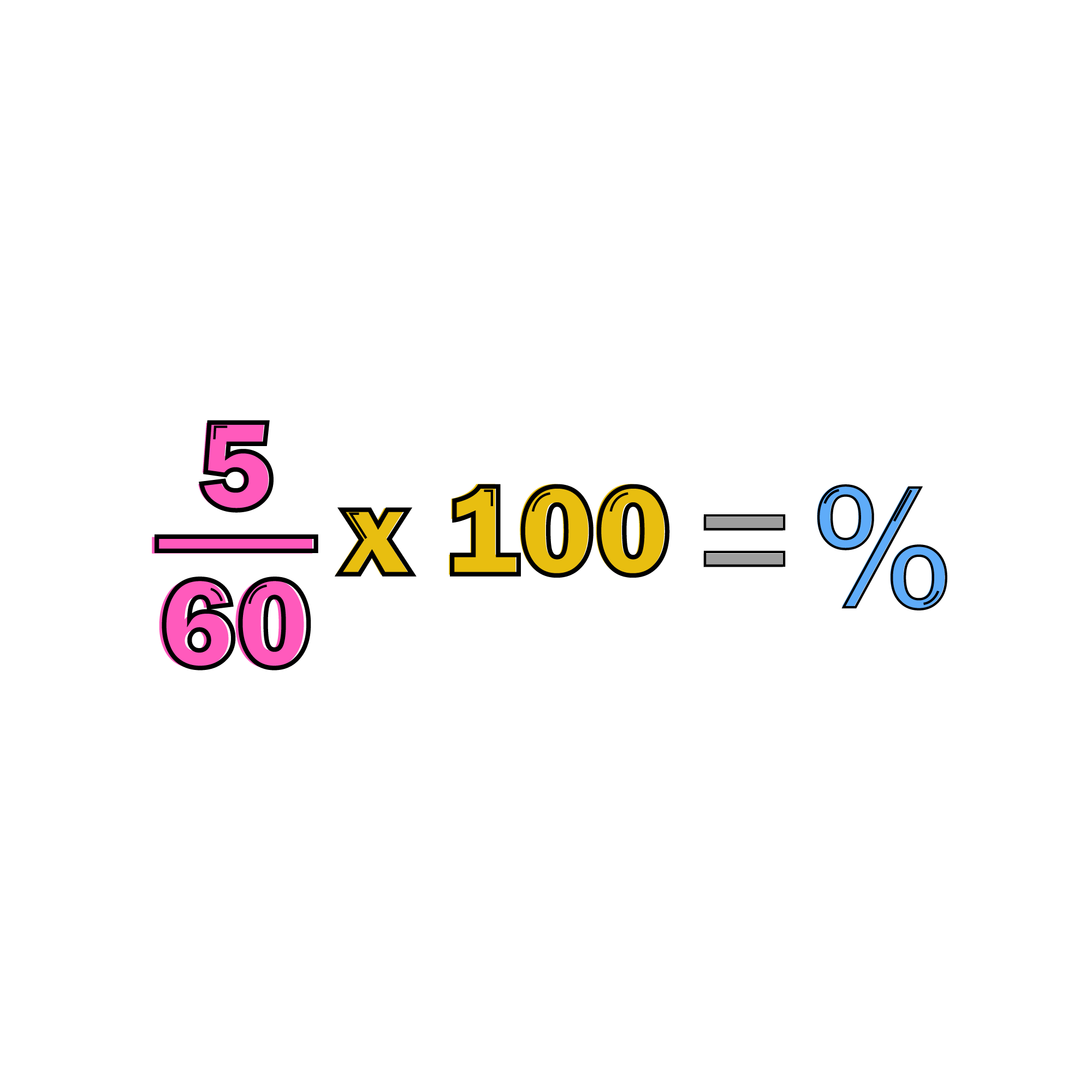SAT Math Basics

## Percentage and Ratios

For some reason, the SAT is absolutely obsessed with percentage problems, and as a result, many students have trouble. The following set of formulas will help eradicate your trouble once and for all.

#### First, let's cover the basics of percentages:

1. 1. If you want to find 35% of a number, simply multiply the number by 0.35. Done.
2. If you want to convert a percentage into a percentage coefficient, simply divide the
number with a percentage sign next to it by 100. For instance, 5% x 100 = 0.05.
3. 50% is not equal to 0.50%. Pay attention to where decimals are located.

Sometimes the SAT will mess with your head by trying to make you reverse or confuse a percentage conversion. If your answer is 60%, then look for either 60% or 0.60 in the answer choices. Not 0.60%. Just keep that in mind – the SAT does this all the time.

With that out of the way, you already know everything you need to know about the simplest of the percentage calculations. However, things always get a little more complicated on the SAT.

## The Ultimate Percentage Change Formula

If you’re looking for the easiest, most standardized way to solve SAT Math Test percentage problems involving any sort of change, here’s the ultimate formula:

(ORIGINAL #) (1.00 +/- PERCENTAGE CHANGE) = FINAL #

But first, let’s explain something: what’s the deal with the “1.00” in the equation? Why do we need that “1.00 plus or minus the coefficient,” instead of just the coefficient?

#### Multiplying a number by 1.00 is the same as finding 100% of the number, which is the same as keeping the number intact. Therefore, we start every percentage-change formula with the 100% already built-in.

• If you’re decreasing a number by 6%, it’s: (1.00 - 0.06), which leaves you with 0.94/94%.
• If you’re increasing a number by 150%, it’s: (1.00 + 1.50), which leaves you with 2.50/250%.

Example: John buys a shirt for 50% off. The price of his new shirt is $70. How much was the shirt before the discount? Original Number = Unknown Percentage Change: Minus 50% Final Number = 70 So, to solve the problem, plug the numbers into the Ultimate Percentage-Change Formula. (Original Price) (1.00 - 0.50) = (70) Original Price = 70 / (0.50) Original price =$140.

Now, you might think that was a very easy problem, and it was. Here’s a tougher that proves how versatile this method is.

## Ratio and Proportions

Ratio-based problems make up a huge share of all SAT Math Test problems, and they can be tricky. Here’s the first way that the SAT will try to force you to slip up.

Suppose you’re asked a question like this: If there are half as many chickens are there are pigs on a farm, then what fraction of the animals on the farm are chickens?

One of the answer choices you’re offered will inevitably be this: 1⁄2. That might seem to make sense. After all, the ratio of chickens to pigs is 1:2, so aren’t half of the animals just chickens?

NO, NO, NO!

THINK ABOUT IT: If there is one chicken that means there must be two pigs. So in total, 1 chicken + 2 pigs = 3 animals. Chickens are 1/3rd of the animals on the farm.

REMEMBER: Ratios are NOT quite the same thing as fractions. They serve a very different role.

From now on, every time you think about, see, or write ratios, imagine an “implied x.”

If you hear that the ratio of girls to boys in a school is 3 to 5, you should see this in your head as 3x : 5x. At this point, you have no idea how many boys or girls there actually are, but that doesn’t matter.

There could be 3 girls or 300,000,000,000.

#### One of three things must happen to determine how many boys and girls are at the school:

1. You’re told what the ratio multiplier, or imaginary x, actually is
2. You’re told the actual number of boys OR girls
3. You’re told the total sum of boys and girls

Once you understand that ratios “MOVE IN GROUPS,” this entire concept will become much easier for you. For example, if the ratio of flies to beetles on your bug farm is 9 to 7, then the ratio of flies to beetles is 9x : 7x.

Since 9x + 7x = 16x, you know that bugs will come in “groups” of 16. You can have 16 bugs, or 32, or 48, or 64 – but you can never have just 7 bugs, or 17, or 31 if you did, then the ratio itself wouldn’t exist.